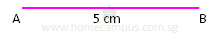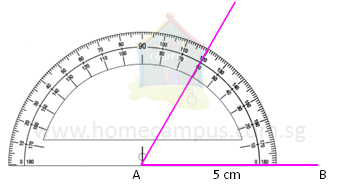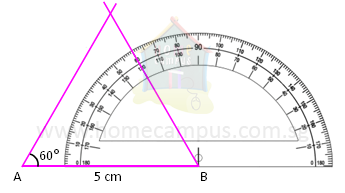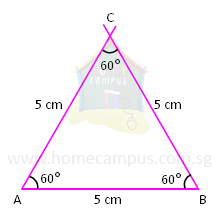## How to Draw and Equilateral Triangle using a Protractor?

Practice Unlimited Questions

#### 1. Draw an equilateral triangle with side 5 cm.

Step 1: Draw a straight line 5 cm long. Call it AB.Step 2: Using a protractor draw an angle of 60° from Point A.Each angle of an equilateral triangle is 60°.
Step 3: Using a protractor draw an angle of 60° from Point B.Step 4: Mark the point where both these lines meet as C.CHECK
1.   Measure the lengths of lines AC and BC.
2.   They should each be equal to 5 cm.
3.   Using a protractor measure ∠ACB.
4.   It should be equal to 60°.
5.   Δ ABC is the required equilateral triangle.
Each side of an equilateral triangle is of the same length.